Graph Plotter 1.2.3Graph Plotter is a powerful utility that will help you plot and analyze different types of graphs.

 Developer: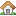Still Soft      software by Still Soft → Price: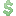25.00  buy → License: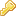Shareware File size: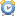0K Language: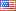OS: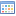Windows Vista (?) Rating: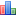0 /5 (0 votes)

Graph Plotter is a powerful utility that will help you plot and analyze different types of graphs. It is easy-to-use and user-friendly.

In addition it can find asymptotes of function, break points, extremums and calculate limit, define integral, derivative of function and more.

Graph Plotter features
• It supports up to 20 graphs. The following graph types are supported:
One variable y(x) and x(y).
Graph tables.
Parametrical x(t) ,y(t).
R(a) in polar coordinates.
f(x,y)=0 graphs of equation.
f(x,y)=0 - graphs of inequalities.
• You can use trigonometrical functions, hyperbolic functions (about 20 different functions) and constants (such as pi,e).
• Plots table functions.
• Plots and Calculates area integral between two curves.
• Calculates definite integral (only for y(x), x(y)).
• Finds Limit (only for y(x), x(y)).
• You can trace a graph.
• Finds and plots vertical, horizontal and oblique asymptotes (only for y(x), x(y)).
• Finds break points (only for y(x), x(y)).
• Finds and plots extremums (only for y(x), x(y)).
• Plots graphs of derivative of function.
• Calculates and draws normal and tangent lines to a function at

tags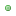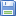Download Graph Plotter 1.2.3

Purchase: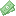Buy Graph Plotter 1.2.3

Authors softwareGraph Plotter 1.2.3
Still Soft

Graph Plotter is a powerful utility that will help you plot and analyze different types of graphs.

Similar softwareGraph Plotter 1.2.3
Still Soft

Graph Plotter is a powerful utility that will help you plot and analyze different types of graphs.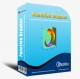Function Grapher 3.9.2
GraphNow

Function Grapher is a easy-to-use software for 2D, 2.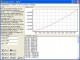Equation graph plotter - EqPlot 1.2
Institute of Mathematics and Statistics

Equation graph plotter software plots 2D graphs from complex mathematical equations.Graph FAWM 1.3
New FAWM Creations

Graph FAWM application is an equation plotter designed for use by students and teachers of mathematics.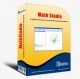Math Studio 3.0
GraphNow

Math Studio offers you an easy-to-use math program for high school teachers and students, that can be used to help study function, sequence of number, inequality, analytic geometry, solid geometry etc.ZigZag 1.3
Palam Software, Inc.

ZigZag is a handy tool to plot and explore math functions.GetData Graph Digitizer 2.22
S. Fedorov

GetData Graph Digitizer is a software that lets you digitize graphs and plots.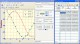Rt-Plot 2.8.10.83
Rt-Science

Rt-Plot is a useful tool that will generate Cartesian X/Y-plots from scientific data.STFMath 2.1
Slavko Ilic

STFMath, technically enhanced math utility, allows you to: draw Cartesian (standard or parametric) and Polar functions, special functions (Gamma, Beta, Fourier, Blancmange) or Integral (with Average preview) in MDI (Multi Documents Interface) supported Graph Mode (open up to 10 independent graph documents in the same window).Aepryus Graph 1.0
Aepryus Software

Aepryus Graph is a 32bit function plotting application capable of 2D and 3D shaded plots of normal and parametric equations, using Cartesian, polar, cylindrical and spherical coordinates.

Other software in this categoryWinNavigator 1.96
WinSoft

WinNavigator is a powerful and easy to use file manager.CuteZIP 2.1 Build 10.26.1
GlobalScape

CuteZIP offers superior ease-of-use, plus powerful security and archiving features for the most versatile compression utility on the market.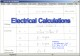Electrical Calculations 2.70.0.4
L.M. Photonics Ltd

Electrical Calculations software provides calculations for busbar and cable ratings, static and bulk power factor correction, motor starting currents and curves, enclosure ventiation, transformer calculations and many metric / imperial conversions and constants.mmSensitivity 2.07
MM&Soft Personal Service

Sensitivity Analysis add-in for Microsoft Excel 97, 98, 2000, and 2001 for Windows.